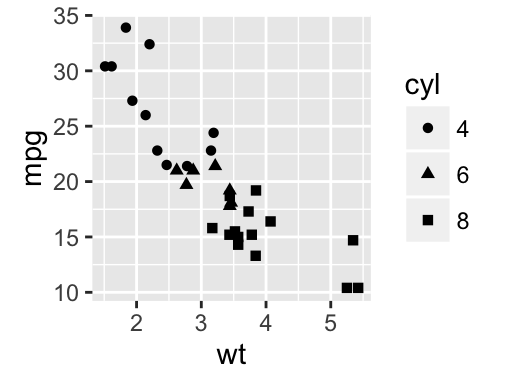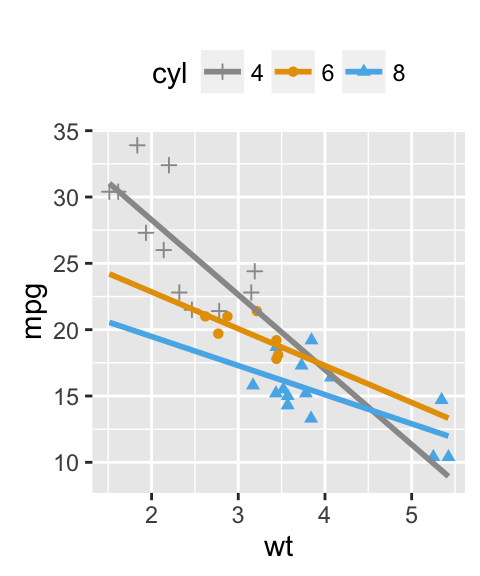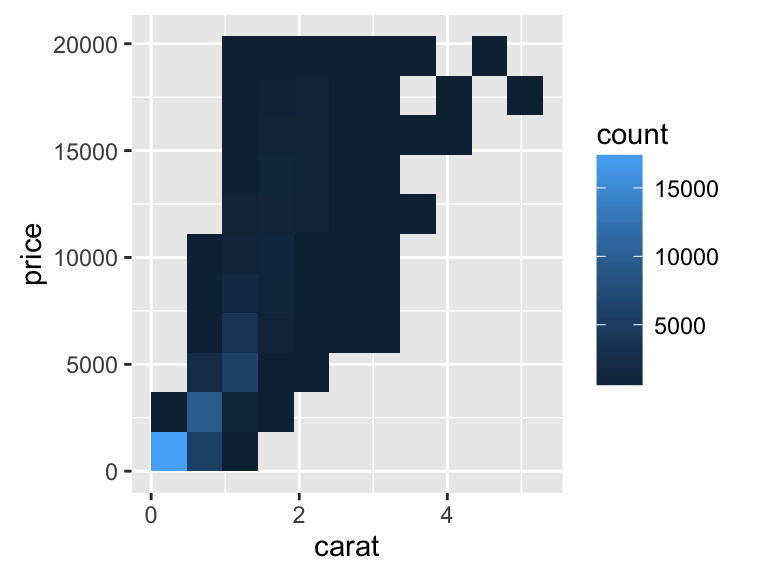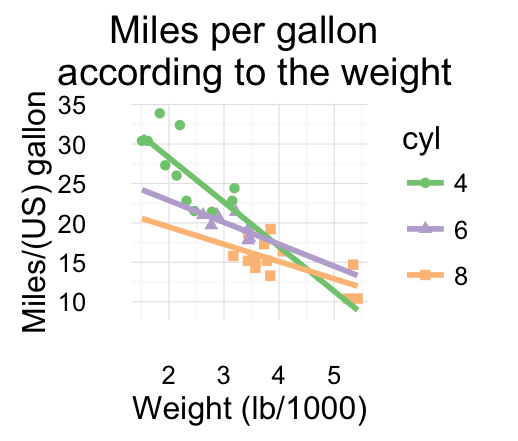# ggplot2 scatter plots : Quick start guide - R software and data visualization

This article describes how create a scatter plot using R software and ggplot2 package. The function geom_point() is used.# Prepare the data

mtcars data sets are used in the examples below.

``````# Convert cyl column from a numeric to a factor variable
mtcars\$cyl <- as.factor(mtcars\$cyl)
``````##                    mpg cyl disp  hp drat    wt  qsec vs am gear carb
## Mazda RX4         21.0   6  160 110 3.90 2.620 16.46  0  1    4    4
## Mazda RX4 Wag     21.0   6  160 110 3.90 2.875 17.02  0  1    4    4
## Datsun 710        22.8   4  108  93 3.85 2.320 18.61  1  1    4    1
## Hornet 4 Drive    21.4   6  258 110 3.08 3.215 19.44  1  0    3    1
## Hornet Sportabout 18.7   8  360 175 3.15 3.440 17.02  0  0    3    2
## Valiant           18.1   6  225 105 2.76 3.460 20.22  1  0    3    1``````

# Basic scatter plots

Simple scatter plots are created using the R code below. The color, the size and the shape of points can be changed using the function geom_point() as follow :

``geom_point(size, color, shape)``
``````library(ggplot2)
# Basic scatter plot
ggplot(mtcars, aes(x=wt, y=mpg)) + geom_point()
# Change the point size, and shape
ggplot(mtcars, aes(x=wt, y=mpg)) +
geom_point(size=2, shape=23)``````Note that, the size of the points can be controlled by the values of a continuous variable as in the example below.

``````# Change the point size
ggplot(mtcars, aes(x=wt, y=mpg)) +
geom_point(aes(size=qsec))``````Read more on point shapes : ggplot2 point shapes

# Label points in the scatter plot

The function geom_text() can be used :

``````ggplot(mtcars, aes(x=wt, y=mpg)) +
geom_point() +
geom_text(label=rownames(mtcars))``````Read more on text annotations : ggplot2 - add texts to a plot

The functions below can be used to add regression lines to a scatter plot :

• geom_smooth() and stat_smooth()
• geom_abline()

Only the function geom_smooth() is covered in this section.

A simplified format is :

``geom_smooth(method="auto", se=TRUE, fullrange=FALSE, level=0.95)``

• method : smoothing method to be used. Possible values are lm, glm, gam, loess, rlm.
• method = “loess”: This is the default value for small number of observations. It computes a smooth local regression. You can read more about loess using the R code ?loess.
• method =“lm”: It fits a linear model. Note that, it’s also possible to indicate the formula as formula = y ~ poly(x, 3) to specify a degree 3 polynomial.
• se : logical value. If TRUE, confidence interval is displayed around smooth.
• fullrange : logical value. If TRUE, the fit spans the full range of the plot
• level : level of confidence interval to use. Default value is 0.95

``````# Add the regression line
ggplot(mtcars, aes(x=wt, y=mpg)) +
geom_point()+
geom_smooth(method=lm)
# Remove the confidence interval
ggplot(mtcars, aes(x=wt, y=mpg)) +
geom_point()+
geom_smooth(method=lm, se=FALSE)
# Loess method
ggplot(mtcars, aes(x=wt, y=mpg)) +
geom_point()+
geom_smooth()``````## Change the appearance of points and lines

This section describes how to change :

• the color and the shape of points
• the line type and color of the regression line
• the fill color of the confidence interval
``````# Change the point colors and shapes
# Change the line type and color
ggplot(mtcars, aes(x=wt, y=mpg)) +
geom_point(shape=18, color="blue")+
geom_smooth(method=lm, se=FALSE, linetype="dashed",
color="darkred")
# Change the confidence interval fill color
ggplot(mtcars, aes(x=wt, y=mpg)) +
geom_point(shape=18, color="blue")+
geom_smooth(method=lm,  linetype="dashed",
color="darkred", fill="blue")``````Note that a transparent color is used, by default, for the confidence band. This can be changed by using the argument alpha : geom_smooth(fill=“blue”, alpha=1)

Read more on point shapes : ggplot2 point shapes

Read more on line types : ggplot2 line types

# Scatter plots with multiple groups

This section describes how to change point colors and shapes automatically and manually.

## Change the point color/shape/size automatically

In the R code below, point shapes, colors and sizes are controlled by the levels of the factor variable cyl :

``````# Change point shapes by the levels of cyl
ggplot(mtcars, aes(x=wt, y=mpg, shape=cyl)) +
geom_point()
# Change point shapes and colors
ggplot(mtcars, aes(x=wt, y=mpg, shape=cyl, color=cyl)) +
geom_point()
# Change point shapes, colors and sizes
ggplot(mtcars, aes(x=wt, y=mpg, shape=cyl, color=cyl, size=cyl)) +
geom_point()````````````# Add regression lines
ggplot(mtcars, aes(x=wt, y=mpg, color=cyl, shape=cyl)) +
geom_point() +
geom_smooth(method=lm)
# Remove confidence intervals
# Extend the regression lines
ggplot(mtcars, aes(x=wt, y=mpg, color=cyl, shape=cyl)) +
geom_point() +
geom_smooth(method=lm, se=FALSE, fullrange=TRUE)``````Note that, you can also change the line type of the regression lines by using the aesthetic linetype = cyl.

The fill color of confidence bands can be changed as follow :

``````ggplot(mtcars, aes(x=wt, y=mpg, color=cyl, shape=cyl)) +
geom_point() +
geom_smooth(method=lm, aes(fill=cyl))``````## Change the point color/shape/size manually

The functions below are used :

• scale_shape_manual() for point shapes
• scale_color_manual() for point colors
• scale_size_manual() for point sizes
``````# Change point shapes and colors manually
ggplot(mtcars, aes(x=wt, y=mpg, color=cyl, shape=cyl)) +
geom_point() +
geom_smooth(method=lm, se=FALSE, fullrange=TRUE)+
scale_shape_manual(values=c(3, 16, 17))+
scale_color_manual(values=c('#999999','#E69F00', '#56B4E9'))+
theme(legend.position="top")

# Change the point sizes manually
ggplot(mtcars, aes(x=wt, y=mpg, color=cyl, shape=cyl))+
geom_point(aes(size=cyl)) +
geom_smooth(method=lm, se=FALSE, fullrange=TRUE)+
scale_shape_manual(values=c(3, 16, 17))+
scale_color_manual(values=c('#999999','#E69F00', '#56B4E9'))+
scale_size_manual(values=c(2,3,4))+
theme(legend.position="top")``````It is also possible to change manually point and line colors using the functions :

• scale_color_brewer() : to use color palettes from RColorBrewer package
• scale_color_grey() : to use grey color palettes
``````p <- ggplot(mtcars, aes(x=wt, y=mpg, color=cyl, shape=cyl)) +
geom_point() +
geom_smooth(method=lm, se=FALSE, fullrange=TRUE)+
theme_classic()
# Use brewer color palettes
p+scale_color_brewer(palette="Dark2")
# Use grey scale
p + scale_color_grey()``````Read more on ggplot2 colors here : ggplot2 colors

# Add marginal rugs to a scatter plot

The function geom_rug() can be used :

``geom_rug(sides ="bl")``

sides : a string that controls which sides of the plot the rugs appear on. Allowed value is a string containing any of “trbl”, for top, right, bottom, and left.

``````# Add marginal rugs
ggplot(mtcars, aes(x=wt, y=mpg)) +
geom_point() + geom_rug()
# Change colors
ggplot(mtcars, aes(x=wt, y=mpg, color=cyl)) +
geom_point() + geom_rug()
# Add marginal rugs using faithful data
ggplot(faithful, aes(x=eruptions, y=waiting)) +
geom_point() + geom_rug()``````# Scatter plots with the 2d density estimation

The functions geom_density_2d() or stat_density_2d() can be used :

``````# Scatter plot with the 2d density estimation
sp <- ggplot(faithful, aes(x=eruptions, y=waiting)) +
geom_point()
sp + geom_density_2d()
sp + stat_density_2d(aes(fill = ..level..), geom="polygon")
sp + stat_density_2d(aes(fill = ..level..), geom="polygon")+Read more on ggplot2 colors here : ggplot2 colors

# Scatter plots with ellipses

The function stat_ellipse() can be used as follow:

``````# One ellipse arround all points
ggplot(faithful, aes(waiting, eruptions))+
geom_point()+
stat_ellipse()
# Ellipse by groups
p <- ggplot(faithful, aes(waiting, eruptions, color = eruptions > 3))+
geom_point()
p + stat_ellipse()
# Change the type of ellipses: possible values are "t", "norm", "euclid"
p + stat_ellipse(type = "norm")``````# Scatter plots with rectangular bins

The number of observations is counted in each bins and displayed using any of the functions below :

• geom_bin2d() for adding a heatmap of 2d bin counts
• stat_bin_2d() for counting the number of observation in rectangular bins
• stat_summary_2d() to apply function for 2D rectangular bins

The simplified formats of these functions are :

``````plot + geom_bin2d(...)
plot+stat_bin_2d(geom=NULL, bins=30)
plot + stat_summary_2d(geom = NULL, bins = 30, fun = mean)``````
• geom : geometrical object to display the data
• bins : Number of bins in both vertical and horizontal directions. The default value is 30
• fun : function for summary

The data sets diamonds from ggplot2 package is used :

``head(diamonds)``
``````##   carat       cut color clarity depth table price    x    y    z
## 1  0.23     Ideal     E     SI2  61.5    55   326 3.95 3.98 2.43
## 2  0.21   Premium     E     SI1  59.8    61   326 3.89 3.84 2.31
## 3  0.23      Good     E     VS1  56.9    65   327 4.05 4.07 2.31
## 4  0.29   Premium     I     VS2  62.4    58   334 4.20 4.23 2.63
## 5  0.31      Good     J     SI2  63.3    58   335 4.34 4.35 2.75
## 6  0.24 Very Good     J    VVS2  62.8    57   336 3.94 3.96 2.48``````
``````# Plot
p <- ggplot(diamonds, aes(carat, price))
p + geom_bin2d()``````Change the number of bins :

``````# Change the number of bins
p + geom_bin2d(bins=10)``````Or specify the width of bins :

``````# Or specify the width of bins
p + geom_bin2d(binwidth=c(1, 1000))``````# Scatter plot with marginal density distribution plot

Step 1/3. Create some data :

``````set.seed(1234)
x <- c(rnorm(500, mean = -1), rnorm(500, mean = 1.5))
y <- c(rnorm(500, mean = 1), rnorm(500, mean = 1.7))
group <- as.factor(rep(c(1,2), each=500))
df <- data.frame(x, y, group)
``````##             x          y group
## 1 -2.20706575 -0.2053334     1
## 2 -0.72257076  1.3014667     1
## 3  0.08444118 -0.5391452     1
## 4 -3.34569770  1.6353707     1
## 5 -0.57087531  1.7029518     1
## 6 -0.49394411 -0.9058829     1``````

Step 2/3. Create the plots :

``````# scatter plot of x and y variables
# color by groups
scatterPlot <- ggplot(df,aes(x, y, color=group)) +
geom_point() +
scale_color_manual(values = c('#999999','#E69F00')) +
theme(legend.position=c(0,1), legend.justification=c(0,1))
scatterPlot
# Marginal density plot of x (top panel)
xdensity <- ggplot(df, aes(x, fill=group)) +
geom_density(alpha=.5) +
scale_fill_manual(values = c('#999999','#E69F00')) +
theme(legend.position = "none")
xdensity
# Marginal density plot of y (right panel)
ydensity <- ggplot(df, aes(y, fill=group)) +
geom_density(alpha=.5) +
scale_fill_manual(values = c('#999999','#E69F00')) +
theme(legend.position = "none")
ydensity``````Create a blank placeholder plot :

``````blankPlot <- ggplot()+geom_blank(aes(1,1))+
theme(plot.background = element_blank(),
panel.grid.major = element_blank(),
panel.grid.minor = element_blank(),
panel.border = element_blank(),
panel.background = element_blank(),
axis.title.x = element_blank(),
axis.title.y = element_blank(),
axis.text.x = element_blank(),
axis.text.y = element_blank(),
axis.ticks = element_blank()
)``````

Step 3/3. Put the plots together:

To put multiple plots on the same page, the package gridExtra can be used. Install the package as follow :

``install.packages("gridExtra")``

Arrange ggplot2 with adapted height and width for each row and column :

``````library("gridExtra")
grid.arrange(xdensity, blankPlot, scatterPlot, ydensity,
ncol=2, nrow=2, widths=c(4, 1.4), heights=c(1.4, 4))``````Read more on how to arrange multiple ggplots in one page : ggplot2 - Easy way to mix multiple graphs on the same page

# Customized scatter plots

``````# Basic scatter plot
ggplot(mtcars, aes(x=wt, y=mpg)) +
geom_point()+
geom_smooth(method=lm, color="black")+
labs(title="Miles per gallon \n according to the weight",
x="Weight (lb/1000)", y = "Miles/(US) gallon")+
theme_classic()
# Change color/shape by groups
# Remove confidence bands
p <- ggplot(mtcars, aes(x=wt, y=mpg, color=cyl, shape=cyl)) +
geom_point()+
geom_smooth(method=lm, se=FALSE, fullrange=TRUE)+
labs(title="Miles per gallon \n according to the weight",
x="Weight (lb/1000)", y = "Miles/(US) gallon")
p + theme_classic()  ``````Change colors manually :

``````# Continuous colors
p + scale_color_brewer(palette="Paired") + theme_classic()
# Discrete colors
p + scale_color_brewer(palette="Dark2") + theme_minimal()
p + scale_color_brewer(palette="Accent") + theme_minimal()``````Read more on ggplot2 colors here : ggplot2 colors

# Infos

This analysis has been performed using R software (ver. 3.2.4) and ggplot2 (ver. 2.1.0)